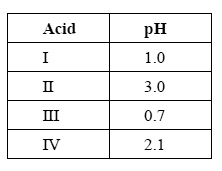Acid-base equilibrium 2008 VCE The following table lists the pH of 0.10 M solutions of four different acids at 25°C.Which one of the four acids listed in the table above has the smallest Ka value? Solution Which acid must have more than one acidic hydrogen per molecule? Give a reason for your answer. Solution Using the concentration and the pH of acid IV, calculate the percentage ionisation of acid IV in the 0.10 M solution. Solution Calculate the value of the ratio [OH–] acid II / [OH–] acid I present in the solutions of acids II and I. Solution Samples of the solutions of acids I and IV are diluted by a factor of 10. The resulting change in pH units would be greater for acid I than for acid IV greater for acid IV than for acid I the same for both acids Give an explanation for your answer. SolutionData sheet is provided for these questions. Methanoic acid is a weak monoprotic acid. Calculate the concentration of a methanoic acid solution that will have the same pH as acid IV. Solution The dissociation of methanoic acid in water is exothermic. If a solution of the acid is heated, will the pH of the solution increase, decrease or remain constant? Give an explanation for your answer. Solution